# Java: Ascii art generator in Java

Ascii art is a technique that uses printable characters from ASCII standard to produce visual art. It had it’s purpose in history when printers lacked graphics ability and it was also used in emails when embedding images was yet not possible. I present you a very simple Ascii Art generator written in Java with configurable font and contrast. Since it was built over a few hours during the weekend, it is not optimal but it was a fun experiment. Down below you can see the code in action and an explanation of how it works.

## The algorithm

The idea is rather simple. First, we create an image of each character we want to use in our ascii art and cache it. Then we go through the original image and for each block of size of the characters we search for the best fit. We do so by first doing some preprocessing of the original image: we convert the image to grayscale and apply a threshold filter. By doing so we get a black and white only contrasted image that we can compare with each character and calculate the difference. We then simply pick the most similar character and do so until the whole image is converted. It is possible to experiment with threshold value to impact contrast and enhance the final result as needed.

A very simple method to accomplish this is to set red, green and blue values to the average of all three:
RED = GREEN = BLUE = (RED + GREEN + BLUE) / 3
If that value is lower than a threshold value, we make it white, otherwise we make it black. Finally, we compare that image with each character pixel by pixel and calculate average error. This is demonstrated in the images and snippet below: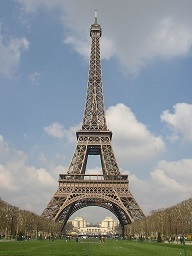``````int r1 = (charPixel >> 16) & 0xFF;
int g1 = (charPixel >> 8) & 0xFF;
int b1 = charPixel & 0xFF;

int r2 = (sourcePixel >> 16) & 0xFF;
int g2 = (sourcePixel >> 8) & 0xFF;
int b2 = sourcePixel & 0xFF;

int thresholded = (r2 + g2 + b2) / 3  THRESHOLD ? 0 : 255;

error = Math.sqrt((r1 - thresholded) * (r1 - thresholded) +
(g1 - thresholded) * (g1 - thresholded) + (b1 - thresholded) * (b1 - thresholded));``````

Since colors are stored in a single integer, we first extract individual color components and perform calculations I explained. Another challenge was to measure character dimensions accurately and to draw them centered. After a lot of experimentation with different methods I finally found this good enough:

``````Rectangle rect = new TextLayout(Character.toString((char) i), fm.getFont(),
fm.getFontRenderContext()).getOutline(null).getBounds();

g.drawString(character, 0, (int) (rect.getHeight() - rect.getMaxY()));``````

Here are a few examples with different font sizes and threshold: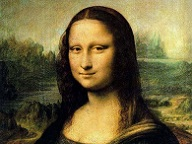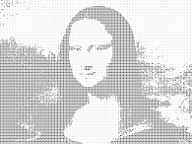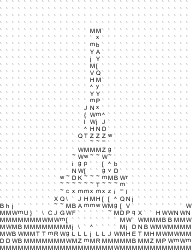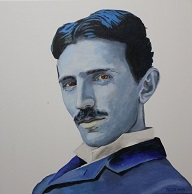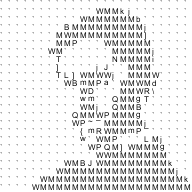CodeProject

This post first appeared on Thoughts About Various Backend Development Topics., please read the originial post: here

# Share the post

Java: Ascii art generator in Java

×